# No. of divisors

How many different divisors have number
$1{3}^{4}\cdot {2}^{4}$
?

Correct result:

n =  25

#### Solution: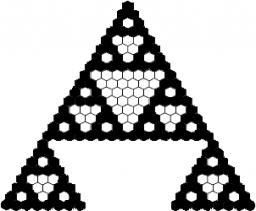We would be pleased if you find an error in the word problem, spelling mistakes, or inaccuracies and send it to us. Thank you!Tips to related online calculators
Do you want to perform natural numbers division - find the quotient and remainder?
Would you like to compute count of combinations?

## Next similar math problems:

• One halfOne half of ? is: ?
• PowersExpress the expression ? as the n-th power of the base 10.
• Digit sumDetermine for how many integers greater than 900 and less than 1,001 has digit sum digit of the digit sum number 1.
• Sum of two primesChristian Goldbach, a mathematician, found out that every even number greater than 2 can be expressed as a sum of two prime numbers. Write or express 2018 as a sum of two prime numbers.
• Divisible by 5How many three-digit odd numbers divisible by 5, which are in place ten's number 3?
• MedalsIn how many ways can be divided gold, silver and bronze medal among 21 contestant?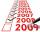Determine the number of integers from 1 to 106 with ending four digits 2006.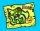There are 4 roads from city A to city B. There are 5 roads from city B to city C. How many different routes can we come from city A to city C via city B?
• Football league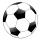In the 5th football league is 10 teams. How many ways can be filled first, second and third place?
• Morse alphabet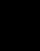Calculate how many words of Morse code to create compiling dashes and dots in the words of one to four characters.
• Coin and die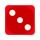Flip a coin and then roll a six-sided die. How many possible combinations are there?
• Area codes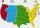How many 6 digit area codes are possible if the first number can't be zero?
• Three-digit integersHow many three-digit natural numbers exist that do not contain zero and are divisible by five?
• Divisors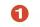Find all divisors of number 493. How many are them?
• Bits, bytes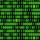Calculate how many different numbers can be encoded in 16-bit binary word?
• How many 4How many 4 digit numbers that are divisible by 10 can be formed from the numbers 3, 5, 7, 8, 9, 0 such that no number repeats?
• Cars plates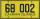How many different licence plates can country have, given that they use 3 letters followed by 3 digits?